Courses

# Test: Chemical Kinetics- 4

## 30 Questions MCQ Test Chemistry Class 12 | Test: Chemical Kinetics- 4

Description
This mock test of Test: Chemical Kinetics- 4 for JEE helps you for every JEE entrance exam. This contains 30 Multiple Choice Questions for JEE Test: Chemical Kinetics- 4 (mcq) to study with solutions a complete question bank. The solved questions answers in this Test: Chemical Kinetics- 4 quiz give you a good mix of easy questions and tough questions. JEE students definitely take this Test: Chemical Kinetics- 4 exercise for a better result in the exam. You can find other Test: Chemical Kinetics- 4 extra questions, long questions & short questions for JEE on EduRev as well by searching above.
QUESTION: 1

### The time for half life period of a certain reaction A → Products is 1 h. When the initial concentration of the reactant 'A' is 2.0 mol L–1, how much time does it take for its concentration to come from 0.50 to 0.25 mol L–1, if it is a zero order reaction?

Solution:

The correct answer is Option A.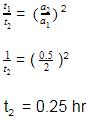QUESTION: 2

### N205 → 2NO2 + O2 When N205 decompose, its t12 does not change with its changing pressure during the reaction, so which one is the correct representation for "pressure of 2NO2" vs lime° during the reaction when initial N205 is equals to Po

Solution:

The correct sentence is option C
Since the half-life period is independent of the changing pressure, the reaction is of the first order.
The integrated rate law is (PN2​O5​​)t​=(P​oN2​O5​)0e−kt
Thus the partial pressure of N2​O5​ decreases exponentially with time and the partial pressure of NO2​ increases exponentially with time.
Hence, the correct graph is represented by option C.

QUESTION: 3

### In a hypothetical reaction A(aq)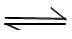2B(aq) + C(aq)    (Ist order decomposition) 'A' is optically active (dextro-rototory) while 'B' and 'C' are optically inactive but 'B' takes part in a titration reaction (fast reaction) with H202. Hence the progress of reaction can be monitored by measuring rotation of plane of plane polarised light or by measuring volume of H202 consumed in titration.  In an experiment the optical rotation was found to be 0 = 30° at t = 20 min and 8 =15° at t= 50 min. from start of the reaction. If the progress would have been monitored by titration method, volume of H202 consumed at t = 30 min. (from start) is 30 ml then volume of H202 consumed at t = 90 min will be:

Solution:

(B) As only A is optically active. So conc. of A at t =20min ∝ 30°

While concentration of A at t= 50min ∝ mc 15°

So conc. has deceased to half oils value in 30 min, so t1/2=30 min.

So volume consumed of H202 at t =30 min =t1/2 ,is according to 50% production of B at t= 90 min. production of B=87.5%(Threehalflives)

So volume consumed =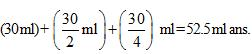QUESTION: 4

The elementary reaction A + B  products has k = 2 x 10-5 M-1 S-1 at a temperature of 27°C. Several experimental runs are carried out using stoichiometric proportion. The reaction has a temperature coefficient value of 2.0. At what temperature should the reaction be carried out if inspite of halving the concentrations,the rate of reaction is desired to be 50% higher than a previous run.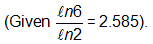Solution: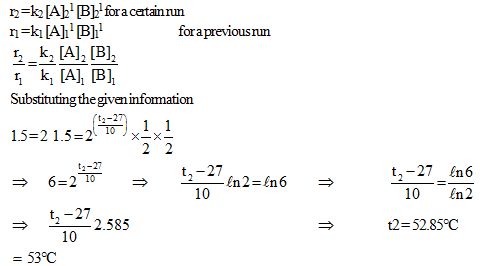QUESTION: 5

At a certain temperature, the first order rate constant k1 is found to be smaller than the second order rate constant k2. If the energy of activation E1 of the first order reaction is greater than energy of activation E2 of the second order reaction, then with increase in temperature.

Solution: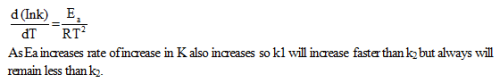QUESTION: 6

For the system

A2(g) + B2(g)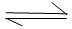2AB(g), AH = – 80 KJ/ mole

If the activation energy for the forward step is 100 kcal /mole. What is the ratio of temperature at which the forward and backward reaction shows the same % change of rate constant per degree rise of temperature ? (1 cal = 4.2 J)

Solution: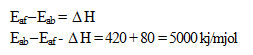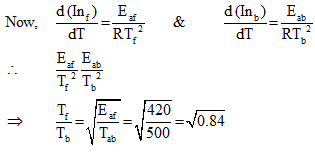QUESTION: 7

All reactions are of Ist order

At time t = ti (ti > 0)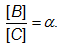Therefore at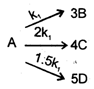time     t = t2 (where t2 > ti)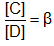which of the following is correct.

Solution: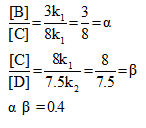QUESTION: 8

In the formation of HBr from H2 & Br2 , following mechanism is observed.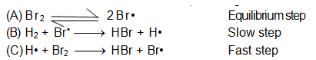Calculate the rate of reaction, it concentration of hydrogen is twice that of bromine and the rate constant is equal to one rutherford. If conc. of bromine is 1 M.

Solution: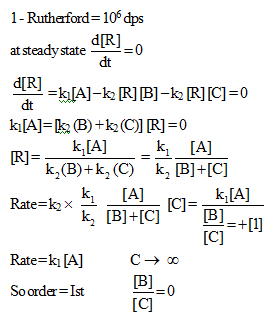QUESTION: 9

A graph between log t112 and log a (abscissa) a being the initial concentration of A in the reaction For reaction A 4 Product, is the rate law is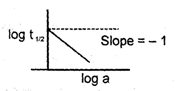Solution: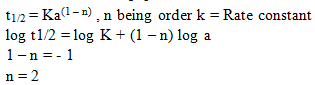QUESTION: 10

The reaction A (g) + B (g)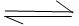C (g) + D (g) is elementry 2nd order reaction. When started with equimolar amounts of A and B, at equilibrium, it is found that the concentration of A is twice that of C. Specific rate for forward reaction is 2 x 10-3 mol-1 Lsec-1. The specific rate constant for backward reaction is :

Solution: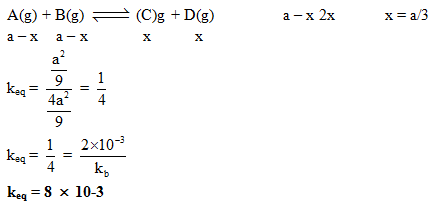QUESTION: 11

For the reaction R – X + OH- ROH + X- The Rate is given of Rate = 5.0x 10-5[R – X [OH-] + 0.20x 10-5[R – X] what percentage R – X Reaction by SN2 mechanism when [OH-–] = 1.0x 10-2 M

Solution: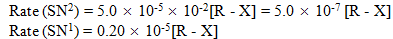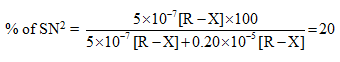QUESTION: 12

For a 1st order reaction (gaseous) (cont. V, T)

a A  → 4 (b 1) B + 1 C

(with b > a) the pressure of the system rose  by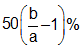in a time of 10 min. The half life of the reaction is therefore.

Solution: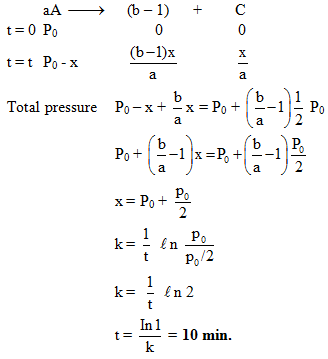QUESTION: 13

For a certain reaction the variation of the rate constant with temperature is given by the equation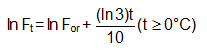The value of tile temperature coefficient of the reaction is

Solution: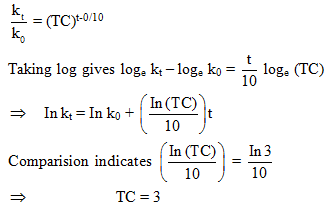QUESTION: 14

For a reaction A + B → C + D if the concentration of A is doubled without altering the concentration of B, the rate gets doubled. If the concentration of B is increased by nine times without altering the concentration of A, the rate gets tripled. The order of the reaction is

Solution: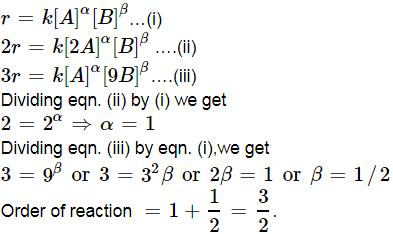QUESTION: 15

A reaction takes place in three steps ; the rate constants are Br k2 and k3.The overall rate constant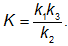If energies of activation are 40 , 30 and 20 kJ, the overall energy of activation is (assumming 'A' to be constant for all )

Solution: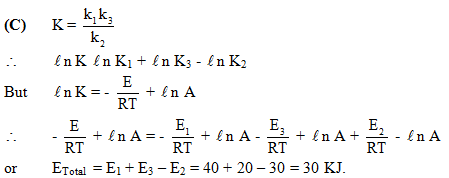QUESTION: 16

The inversion of cane sugar proceeds with half life of 600 minute at pH = 5 for any concentration of sugar, However at pH = 6, the half life changes to 60 minute, The rate law expression for sugar inversion can be written as

Solution: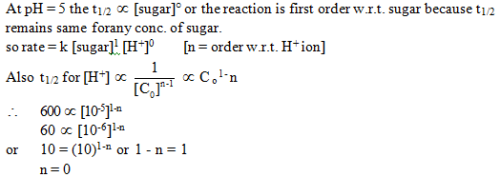QUESTION: 17

Some graph are sketch for the reaction AB (assuming different orders).Where 'α' represent the degree of dissociation.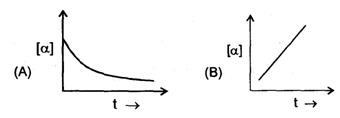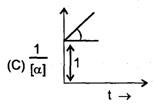The order of reaction is respectively.

Solution: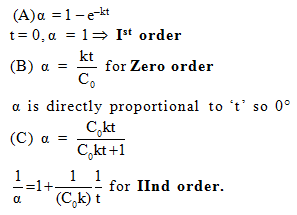QUESTION: 18

Compounds A and B react with a common reagent with first order kinetics in both cases. If 99% of A must react before 1% of B has reacted. What is the minimum ratio for their respective rate constants?

Solution: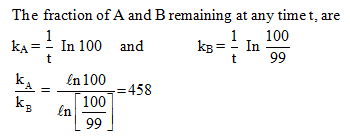QUESTION: 19

The activity per ml of a solution of radioactive substance is x. How much water be added to 200 ml of this solution so that the acitivity falls to x/20 per ml after 4 half-lives ?

Solution: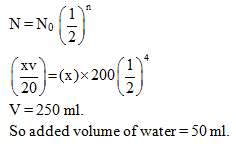QUESTION: 20

A hypothetical reaction :

A2 + B2 → 2AB Follows mechanism as given below :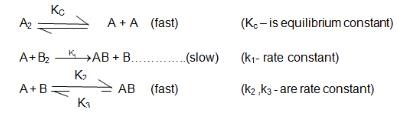The order of overall reaction is

Solution: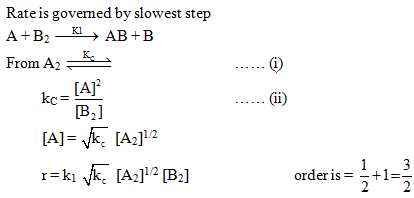QUESTION: 21

For the decomposition of H202(aq) it was found that Vo2 (t =15 min.) was 100 mL (at 0°C and 1 atm) while Vo2(maximum) was 200 mL (at 0°C and 2 atm). If the same reaction had been followed by the titration method and if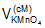(t = 0) had been 40 mL, what would(t = 15 min) have been ?

Solution: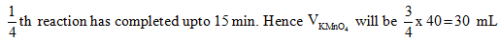QUESTION: 22

The high temperature (z, 1200K) decomposition of CH3COOH(g) occurs as follows as per simultaneous 1St order reactions.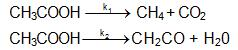What would be the % of CH4 by mole in the product mixture (excluding CH3COOH)?

Solution: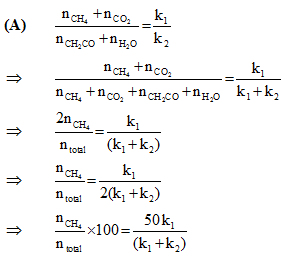QUESTION: 23

For the system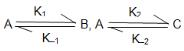which was started with only A the equilibrium concentration [A]eq is correctly related to [B]eq and [C]eq. as:

Solution:
QUESTION: 24

A certain reaction A -3 B follows the given concentration (Molarity)-time graph. Which of the following statement is true?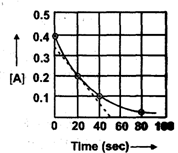Solution: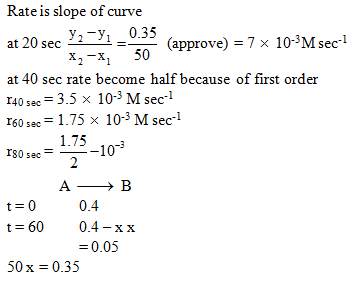QUESTION: 25

The activation energies of two reactions are Ea and Ea with Ea > EaIf temperature of the reacting systems is increased from T1 to T2, predict which of the following alternatives

Solution:

The correct  answer is option B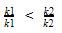Greater the activation energy of a reaction, greater is the temperature dependence of rate constant of reaction.

QUESTION: 26

A simple mechanism for enzyme-catalyzed reaction is given by the following of  equations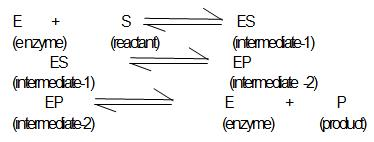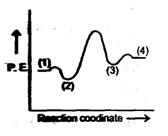This is known as the Michaleis-mechanism. The potential energy diagram is shown in the figure. Which of the following sets of identifications is correct ? (Assume that the temperature and pressure are constant).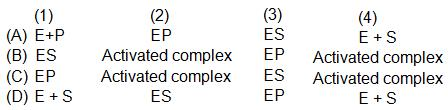Solution:

(1) E+S (2) ES (3) EP (4) E+P
Intermediate and product have less potential energy than activated complex.

QUESTION: 27

Statement-1 : If the activation energy of reaction is zero temperature will have no effect on the rate constant.

Statement-2 : Lower the activation energy fasten is the reaction.

Solution:

According to arrhenius equation K = AeEa/RT when Ea = 0, K = A.

QUESTION: 28

Statement-1 : If the order of reaction is zero than degree of dissociation will be independent upon initial concentration.

Statement-2 : The degree of dissociation of Zero order reaction is given by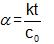Solution:

For zero order reactions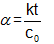They depend upon initial concentration

QUESTION: 29

Statement-1 : For a reaction A(g) → B(g)

— re = 2.5 PA           at    400K

— re = 2.5 PA           at    600K

activation energy is 4135 J/mol.

Statement-2 : Since for any reaction, values of rate constant at two different temp is same therefore activation energy of the reaction is zero.

Solution: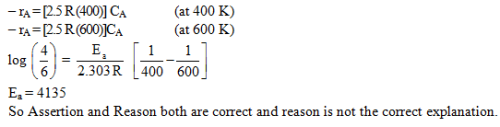QUESTION: 30

Statement-1 : For A+ 2B → C (rate = K [A]1[B]°), the half life time of reaction is only defined when conch of A and B are in stoichometric ratio

Statement-2 : For above given order half life of reaction is directly proportional to conch of A and not to conch of B due to its zero order.

Solution:

The correct answer is option C
If the concentration of B is much lower than the stoichiometric concentration, then there will not be sufficient  quantity of B to react with A and the half life period cannot be defined. Hence, the assertion is correct.
Since the reaction is first order with respect to A, the half life is independent of the concentration of A.